Question

Let f(x,y) = (x" + 2?y?)!. compute all second-order partial derivatives of fat (0,0), if they...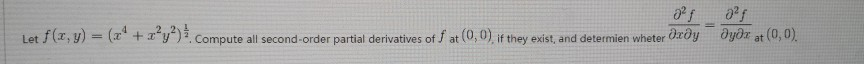Let f(x,y) = (x" + 2?y?)!. compute all second-order partial derivatives of fat (0,0), if they exist, and determien wheter dæðyəyər at (0,0).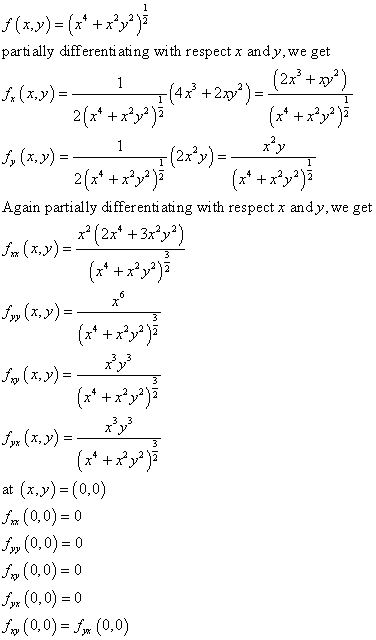Earn Coins

Coins can be redeemed for fabulous gifts.

Similar Homework Help Questions
• Exercice 2 (5pts) Let f given by f(x, y) Isinyif (x, y) (0,0) and f(0,0) 0...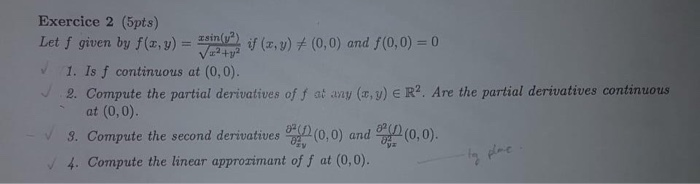Exercice 2 (5pts) Let f given by f(x, y) Isinyif (x, y) (0,0) and f(0,0) 0 1V224 1. Is f continuous at (0,0). 2. Compute the partial derivatives of f at any (x, y) E R2. Are the partial derivatives continuous (0,0). at (0,0) (0,0) and 3. Compute the second derivatives 4. Compute the linear approzimant of f at (0,0). Exercice 2 (5pts) Let f given by f(x, y) Isinyif (x, y) (0,0) and f(0,0) 0 1V224 1. Is f...

• Find all second order partial derivatives of f(x, y) = (x² +3y)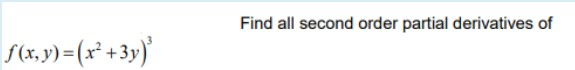Find all second order partial derivatives of f(x, y) = (x² +3y)

• *Let f : R2 -R be given by z, y)(0,0 r, y)- 2y and f(0,0) =...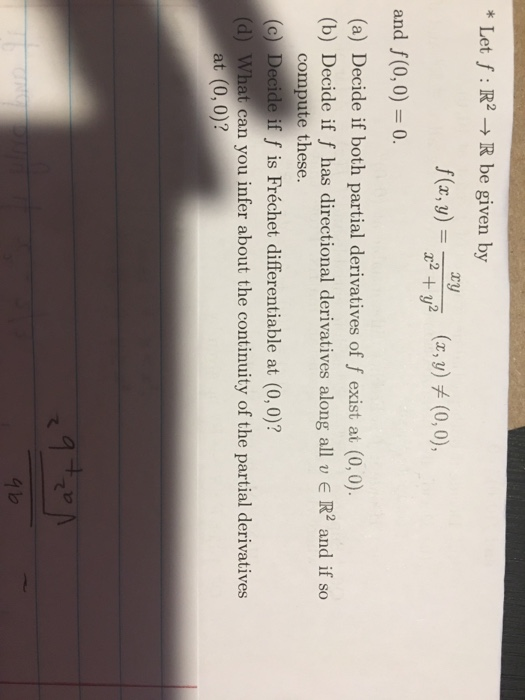*Let f : R2 -R be given by z, y)(0,0 r, y)- 2y and f(0,0) = 0. (a) Decide if both partial derivatives of f exist at (0, 0) (b) Decide if f has directional derivatives along all v R2 and if so compute these. (c) Decide if f is Fréchet differentiable at (0, 0)? (d) What can you infer about the continuity of the partial derivatives at (0, 0)? て

• 5. (a) Suppose f is any function with continuous second-order partial derivatives such that f(0,0) =...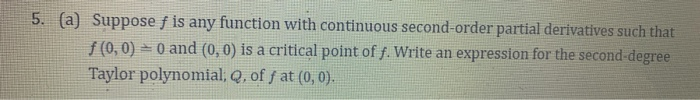5. (a) Suppose f is any function with continuous second-order partial derivatives such that f(0,0) = 0 and (0,0) is a critical point of f. Write an expression for the second-degree Taylor polynomial, Q, of f at (0,0).

• Find all the first and second order. partial derivatives of f(x, y) = 8 sin(2x +...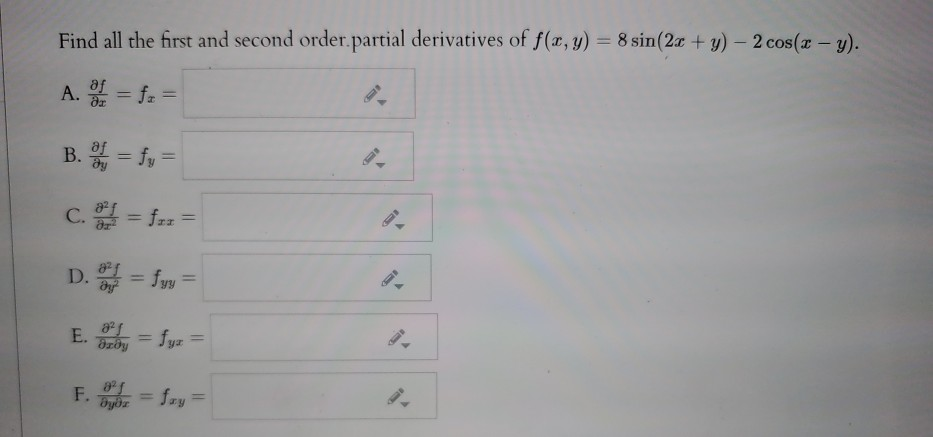Find all the first and second order. partial derivatives of f(x, y) = 8 sin(2x + y) - 2 cos(x - y). A. SI = fr = B. = fy = c. = f-z = D. = fyy = E. By = fyz = F. = Sxy=

• Question 2 (20 points): Consider the functions f(x, y)-xe y sin y and g(x, y)-ys 1. Show f is differentiable in its domain 2. Compute the partial derivatives of g at (0,0) 3. Show that g is not d...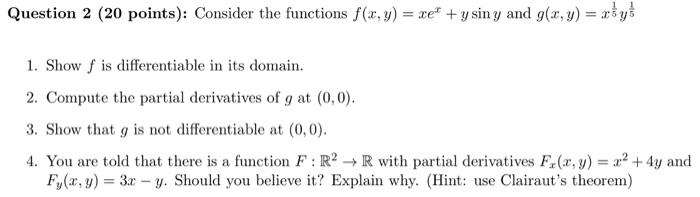Question 2 (20 points): Consider the functions f(x, y)-xe y sin y and g(x, y)-ys 1. Show f is differentiable in its domain 2. Compute the partial derivatives of g at (0,0) 3. Show that g is not differentiable at (0,0) 4. You are told that there is a function F : R2 → R with partial derivatives F(x,y) = x2 +4y and Fy(x, y 3x - y. Should you believe it? Explain why. (Hint: use Clairaut's theorem) Question 2...

• Find the first-order partial derivatives (fr. f,) and second-order partial derivatives (fxxıfyy, fxy, fyx) of the...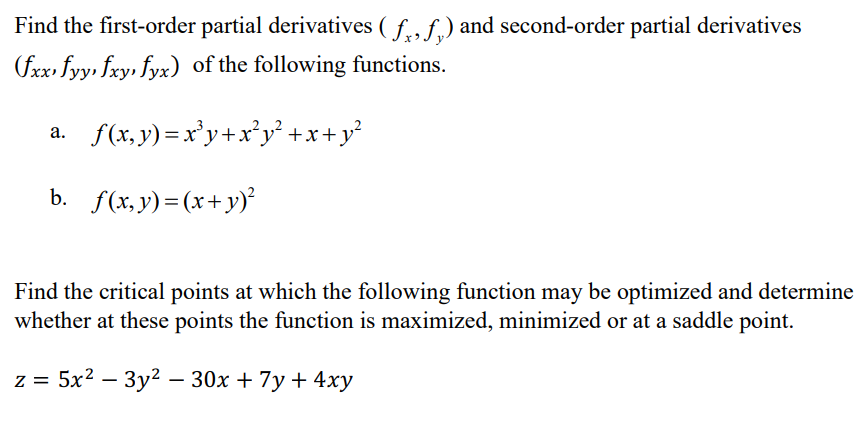Find the first-order partial derivatives (fr. f,) and second-order partial derivatives (fxxıfyy, fxy, fyx) of the following functions. a. f(x,y)=x’y+x’y? +x+y? b. f(x, y) = (x + y)? Find the critical points at which the following function may be optimized and determine whether at these points the function is maximized, minimized or at a saddle point. z = 5x2 – 3y2 – 30x + 7y + 4xy

• 2. Consider the following function: Compute each of the following: Hint: There is probably a better way to compute these than to just mindlessly compute all ot partial derivatives in the order gi...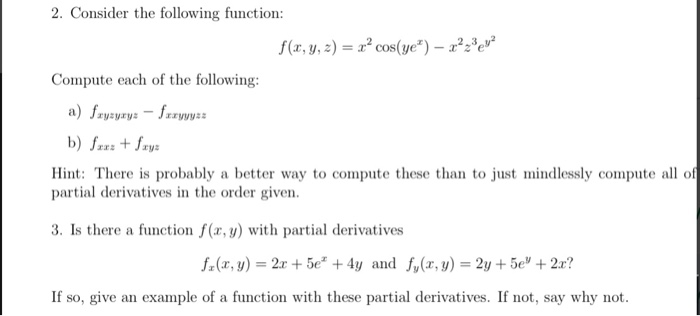2. Consider the following function: Compute each of the following: Hint: There is probably a better way to compute these than to just mindlessly compute all ot partial derivatives in the order given 3. Is there a function f(x, y) with partial derivatives f, (z, y) = 2r + 5e" + 4y and f,(x, y) = 2y + 5e" + 2x? If so, give an example of a function with these partial derivatives. If not, say why not 2. Consider...

• Problem 8. (1 point) (1 pt) Calculate all four second-order partial derivatives of f(x,y) = 2x²y...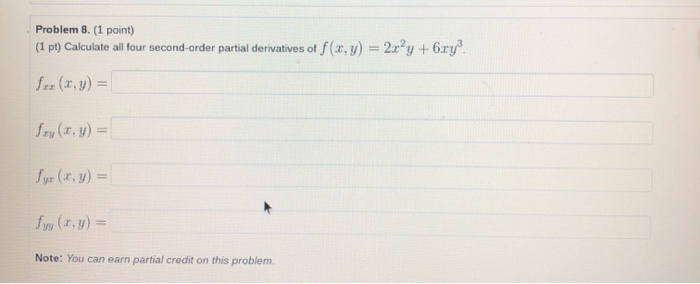Problem 8. (1 point) (1 pt) Calculate all four second-order partial derivatives of f(x,y) = 2x²y +6.ry? for (x,y) = Szy(x, y) = Syr (x, y) = fry (x. y) = Note: You can earn partial credit on this problem.

• . M Compute all the first and second partial derivatives of the function f(x,t) = 2...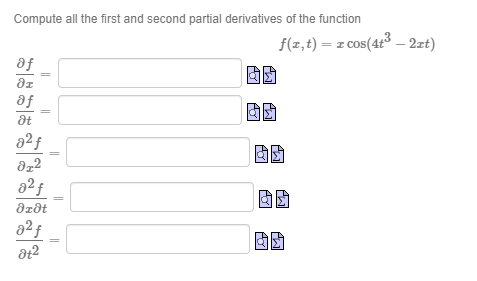. M Compute all the first and second partial derivatives of the function f(x,t) = 2 COS(4+3 – 27t) af ar af at 22 a22 TO M PO 02f δεδε 02f at2 M